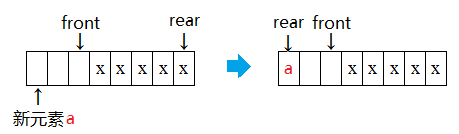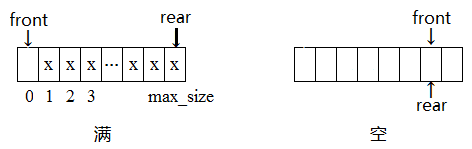# 队列 Queue 的循环数组实现

队列（queue）是一种基本的线性结构，其特点是先进先出（First In First Out, FIFO）。队列可以用数组或链表实现。当用数组实现时，为了提高空间利用率，数组要“循环使用”。如下图所示。用循环数组的方式实现时，为了方便地判断队列是否为空或者满，可以采用以下方式：

1) 设队列最大容量为 max_size，那么要开一个长度为 max_size+1的数组。因为，队列为0, 1, ..., max_size 个元素共 max_size+1 种状态。

2) 如上图所示， 设 rear 为当前队列尾部元素在数组中的下标位置，front 为当前队列头部元素的逻辑上前一个位置的数组下标，存储队列元素的数组下标范围 0 ~ max_size，则：

初始时，front = rear = 0。

当有元素入队时，先判断是否满，不满则更新尾部位置 rear = (rear + 1) % (max_size + 1)，然后将新入队元素加到数组下标为 rear 处。

当有元素出队时，先判断是否空，不空则更新头部位置 front = (front + 1) % (max_size + 1)，然后该 front 位置元素为出队元素。

队列为满的条件是：(rear + 1) % (max_size + 1) == front。

队列为空的条件是：front == rear。队列循环数组实现（C++）

using namespace std;

const int MAXSIZE = 100000;

/* 用循环数组实现的队列 */
template <class T>
class Queue
{
private:
int max_size; // 队列最大容量
int front;    // 当前队头的数组下标
int rear;     // 当前队尾的数组下标
T * array;    // 实际存放队列元素的数组

public:
Queue()  // 默认构造函数
{
max_size = MAXSIZE;
front = rear = 0;
array = new T[max_size+1];
}

Queue(int _max_size)    // 指定最大队列容量的构造函数
{
max_size = _max_size < MAXSIZE ? _max_size : MAXSIZE;
front = rear = 0;
array = new T[max_size+1];
}

~Queue() { delete [] array; }    // 析构函数

bool full()    // 判断当前队列是否满
{
return (rear + 1) % (max_size + 1) == front;
}

bool empty()   // 判断当前队列是否为空
{
return front == rear;
}

int push(T & e)    // 将元素e入队，若成功则返回当前队尾位置，否则返回-1
{
if ( this->full() )
return -1;
rear = (rear + 1) % (max_size + 1);
array[rear] = e;
return rear;
}

T & query(int pos)  // 返回队列中位置为pos的元素，pos>=0, pos=0为队头。
{
pos = (front + pos + 1) % (max_size + 1);
return array[pos];
}

T pop()    // 得到当前队尾元素，如果队列为空，则返回的元素无意义
{
if ( this->empty() )
return array[front];
front = (front + 1) % (max_size + 1);
return array[front];
}
}; 

©️2019 CSDN 皮肤主题: 编程工作室 设计师: CSDN官方博客